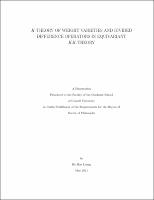## K-Theory Of Weight Varieties And Divided Difference Operators In Equivariant Kk-Theory##### Abstract
This thesis consists of two chapters. In the first chapter, we compute the K theory of weight varieties by using techniques in Hamiltonian geometry. In the second chapter, we construct a set of divided difference operators in equivariant KK -theory. Let T be a compact torus and (M, [omega] ) a Hamiltonian T -space. In Chapter 1, we give a new proof of the K -theoretic analogue of the Kirwan surjectivity theorem in symplectic geometry (see [HL1]) by using the equivariant version of the Kirwan map introduced in [G2]. We compute the kernel of this equivariant Kirwan map. As an application, we find the presentation of the K -theory of weight varieties, which are the symplectic quotients of complete flag varieties G/T , as the quotient ring of the T -equivariant K -theory of flag varieties by the kernel of the Kirwan map, where G is a compact, connected and simply-connected Lie group. Demazure [D1], [D2], [D3] defined a set of isobaric divided difference operators on the representation ring R(T ). It can be seen as a decomposition of the classical Weyl character formula. In [HLS], Harada, Landweber and Sjamaar defined an analogous set of divided difference operators on the equivariant K -theory. In Chapter 2, we explicitly define these operators in the setting of equivariant KK theory first defined by Kasparov [K1], [K2]. It is a generalization of the results in [D3] and [HLS]. Due to the elegance and generality of equivariant KK -theory, some interesting applications of the result will also be given.
2011-05-31
##### Keywords
Symplectic Geometry; Operator Algebras; Divided difference operators; KK-theory
Sjamaar, Reyer
Holm, Tara S.
Knutson, Allen
Mathematics
##### Degree Name
Ph. D., Mathematics
##### Degree Level
Doctor of Philosophy
##### Types
dissertation or thesis